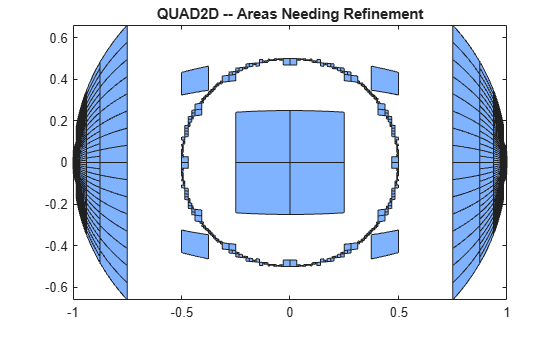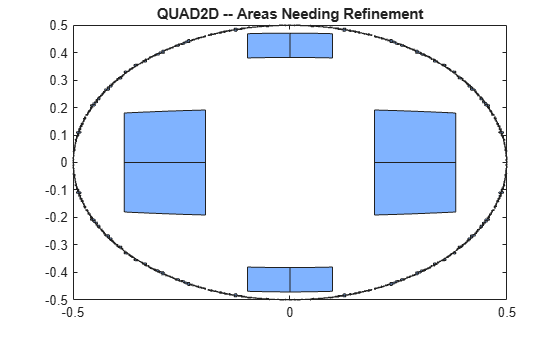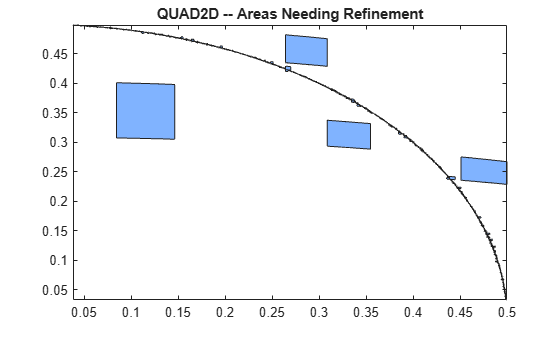Numerically evaluate double integral — tiled method

## Syntax

``q = quad2d(fun,a,b,c,d)``
``q = quad2d(fun,a,b,c,d,Name,Value)``
``[q,E] = quad2d(___)``

## Description

example

````q = quad2d(fun,a,b,c,d)` approximates the integral of `fun(x,y)` over the planar region $a\le x\le b$ and $c\left(x\right)\le y\le d\left(x\right)$. The bounds `c` and `d` can each be scalars or function handles.```

example

````q = quad2d(fun,a,b,c,d,Name,Value)` specifies additional options with one or more `Name,Value` pair arguments. For example, you can specify `'AbsTol'` and `'RelTol'` to adjust the error thresholds that the algorithm must satisfy.```
````[q,E] = quad2d(___)` also returns an approximate upper bound on the absolute error, E = | q - I |, where I is the exact value of the integral.```

## Examples

collapse all

Integrate

`$y\mathrm{sin}\left(x\right)+x\mathrm{cos}\left(y\right)$`

over $-\pi \le x\le 2\pi$ and $0\le y\le \pi$.

```fun = @(x,y) y.*sin(x)+x.*cos(y); Q = quad2d(fun,pi,2*pi,0,pi)```
```Q = -9.8696 ```

Compare the result to the true value of the integral, $-{\pi }^{2}$.

`-pi^2`
```ans = -9.8696 ```

Integrate the function

`${\left[{\left(x+y\right)}^{1/2}{\left(1+x+y\right)}^{2}\right]}^{-1}$`

over the region $0\le x\le 1$ and $0\le y\le 1-x$. This integrand is infinite at the origin (0,0), which lies on the boundary of the integration region.

```fun = @(x,y) 1./(sqrt(x + y) .* (1 + x + y).^2 ); ymax = @(x) 1 - x; Q = quad2d(fun,0,1,0,ymax)```
```Q = 0.2854 ```

The true value of the integral is $\pi /4-1/2$.

`pi/4 - 0.5`
```ans = 0.2854 ```

`quad2d` begins by mapping the region of integration to a rectangle. Consequently, it may have trouble integrating over a region that does not have four sides or has a side that cannot be mapped smoothly to a straight line. If the integration is unsuccessful, some helpful tactics are leaving `Singular` set to its default value of `true`, changing between Cartesian and polar coordinates, or breaking the region of integration into pieces and adding the results of integration over the pieces.

For instance:

```fun = @(x,y)abs(x.^2 + y.^2 - 0.25); c = @(x)-sqrt(1 - x.^2); d = @(x)sqrt(1 - x.^2); quad2d(fun,-1,1,c,d,'AbsTol',1e-8,... 'FailurePlot',true,'Singular',false);```
```Warning: Reached the maximum number of function evaluations (2000). The result fails the global error test. ```The failure plot shows two areas of difficulty, near the points `(-1,0)` and `(1,0)` and near the circle ${x}^{2}+{y}^{2}=0.25$.

Changing the value of `Singular` to `true` will cope with the geometric singularities at `(-1,0)` and `(1,0)`. The larger shaded areas may need refinement but are probably not areas of difficulty.

```Q = quad2d(fun,-1,1,c,d,'AbsTol',1e-8, ... 'FailurePlot',true,'Singular',true);```
```Warning: Reached the maximum number of function evaluations (2000). The result passes the global error test. ```From here you can take advantage of symmetry:

```Q = 4*quad2d(fun,0,1,0,d,'Abstol',1e-8,... 'Singular',true,'FailurePlot',true)```
```Q = 0.9817 ```

However, the code is still working very hard near the singularity. It may not be able to provide higher accuracy:

```Q = 4*quad2d(fun,0,1,0,d,'Abstol',1e-10,... 'Singular',true,'FailurePlot',true);```
```Warning: Reached the maximum number of function evaluations (2000). The result passes the global error test. ```At higher accuracy, a change in coordinates may work better.

```polarfun = @(theta,r) fun(r.*cos(theta),r.*sin(theta)).*r; Q = 4*quad2d(polarfun,0,pi/2,0,1,'AbsTol',1e-10);```

It is best to put the singularity on the boundary by splitting the region of integration into two parts:

```Q1 = 4*quad2d(polarfun,0,pi/2,0,0.5,'AbsTol',5e-11); Q2 = 4*quad2d(polarfun,0,pi/2,0.5,1,'AbsTol',5e-11); Q = Q1 + Q2;```

## Input Arguments

collapse all

Function to integrate, specified as a function handle. The function `Z = fun(X,Y)` must accept 2-D matrices `X` and `Y` of the same size and return a matrix `Z` of corresponding values. Therefore, the function must be vectorized (that is, you must use elementwise operators such as `.^` instead of matrix operators such as `^`). The inputs and outputs of the function must be either single or double precision.

Example: `@(x,y) x.^2 - y.^2`

Data Types: `function_handle`

x limits of integration, specified as scalars.

Data Types: `single` | `double`
Complex Number Support: Yes

y limits of integration, specified as scalars or function handles. Each limit can be specified as a scalar or a function handle. If the limits are specified as function handles, then they are functions of the x limit of integration ```ymin = @x c(x)``` and `ymax = @(x) d(x)`. The function handles `ymin` and `ymax` must accept matrices and return matrices of the same size with the corresponding values. The inputs and outputs of the functions must be either single or double precision.

Data Types: `single` | `double` | `function_handle`
Complex Number Support: Yes

### Name-Value Pair Arguments

Specify optional comma-separated pairs of `Name,Value` arguments. `Name` is the argument name and `Value` is the corresponding value. `Name` must appear inside quotes. You can specify several name and value pair arguments in any order as `Name1,Value1,...,NameN,ValueN`.

Example: `quad2d(@(x,y) x.*y.^2, 0, 1, 0, 2, 'AbsTol',1e-3)` specifies the absolute tolerance for the integration as `1e-3`.

Absolute error tolerance, specified as the comma-separated pair consisting of `'AbsTol'` and a scalar.

`quad2d` attempts to satisfy ```ERRBND <= max(AbsTol,RelTol*|Q|)```. This is absolute error control when `|Q|` is sufficiently small and relative error control when `|Q|` is larger. A default tolerance value is used when a tolerance is not specified. The default value of `AbsTol` is 1e-5. The default value of `RelTol` is `100*eps(class(Q))`. This is also the minimum value of `RelTol`. Smaller `RelTol` values are automatically increased to the default value.

Relative error tolerance, specified as the comma-separated pair consisting of `'RelTol'` and a scalar.

`quad2d` attempts to satisfy ```ERRBND <= max(AbsTol,RelTol*|Q|)```. This is absolute error control when `|Q|` is sufficiently small and relative error control when `|Q|` is larger. A default tolerance value is used when a tolerance is not specified. The default value of `AbsTol` is 1e-5. The default value of `RelTol` is `100*eps(class(Q))`. This is also the minimum value of `RelTol`. Smaller `RelTol` values are automatically increased to the default value.

Maximum number of evaluations of `fun`, specified as the comma-separated pair consisting of `'MaxFunEvals'` and a scalar. Use this option to limit the number of times `quad2d` evaluates the function `fun`.

Toggle to generate failure plot, specified as the comma-separated pair consisting of `'FailurePlot'` and a numeric or logical `1` (`true`) or `0` (`false`). Set `FailurePlot` to `true` or `1` to generate a graphical representation of the regions needing further refinement when `MaxFunEvals` is reached. No plot is generated if the integration succeeds before reaching `MaxFunEvals`. The failure plot contains (generally) 4-sided regions that are mapped to rectangles internally. Clusters of small regions indicate the areas of difficulty in the integration.

Toggle to transform boundary singularities, specified as the comma-separated pair consisting of `'Singular'` and a numeric or logical `1` (`true`) or `0` (`false`). By default, `quad2d` employs transformations to weaken boundary singularities for better performance. Set `'Singular'` to `false` or `0` to turn these transformations off, which can provide a performance benefit on some smooth problems.

## Output Arguments

collapse all

Calculated integral, returned as a scalar.

Error bound, returned as a scalar. The error bound provides an upper bound on the error between the calculated integral q and the exact value of the integral I such that E = | q - I |.

 L.F. Shampine, "Matlab Program for Quadrature in 2D." Applied Mathematics and Computation. Vol. 202, Issue 1, 2008, pp. 266–274.